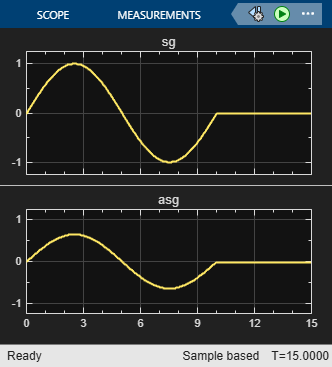# Using an External Function in a Test Sequence Block

This example shows how to call an externally-defined function from the Test Sequence block. The provided function, `Attenuate.m`, is defined in a script on the MATLAB® path and is called from the test sequence. The `Attenuate.m` function is:

` function[y] = Attenuate(x)`

` y = 0.65*x;`

` end`

#### Open the `TestSeqOutputFunctions` model

```model = 'TestSeqOutputFunctions'; open_system(model)```#### Open the Test Sequence block

In the model, double-click the Test Sequence block, Calling ext functions. The ReducedSine step in the Test Sequence block uses the external function, `Attenuate`, to reduce the amplitude of the signal `sg`.#### Simulate the model

Simulate the model and view the output signal `sg` and attenuated signal `asg` in the Scope2 block.

```sim(model) open_system([model '/Scope2'])````close_system(model,0)`